Vibroengineering PROCEDIA

Published: 02 April 2020

Xiabing Yue1
Yapeng Niu2
1, 2College of Highway, Chang’an University, Xi’an 710064, China
1Zhong Jiao Tong Li Construction Co., Ltd, Xi’an 710064, China
Corresponding Author:
Yapeng Niu
Views 361

#### Abstract

This paper described a continuous load one-dimensional ${K}_{0}$ consolidation apparatus developed and improved, conducted an axial cyclic loading and unloading test of saturated soft clay. It can be known from the experimental comparison that during the unloading period, a stress strain curve of saturated soft clay is made hyperbolic. During the reloading period, when the loading quantity is less than the unloading quantity, the curve is hyperbolic, and when the loading quantity is greater than unloading quantity the curve is a straight line which keeps hardening. The plastic deformation and elastic deformation of test samples produced in the test have linear relation with the unloading level. On the basis of the original constitutive relation, axial circulation loading and unloading constitutive equation have been established using the loading axial stresses, initial tangent modulus, maximum unloading axial strain and its initial secant modulus as parameters. The reliability of equations was verified as well. The analysis of various plastic hysteresis reveals that as the unloading stress level increases, the hysteresis increases too and conforms to the quadratic curve relationship. This provides a certain engineering practice value for the strength and deformation characteristics of soft soil under cyclic loading.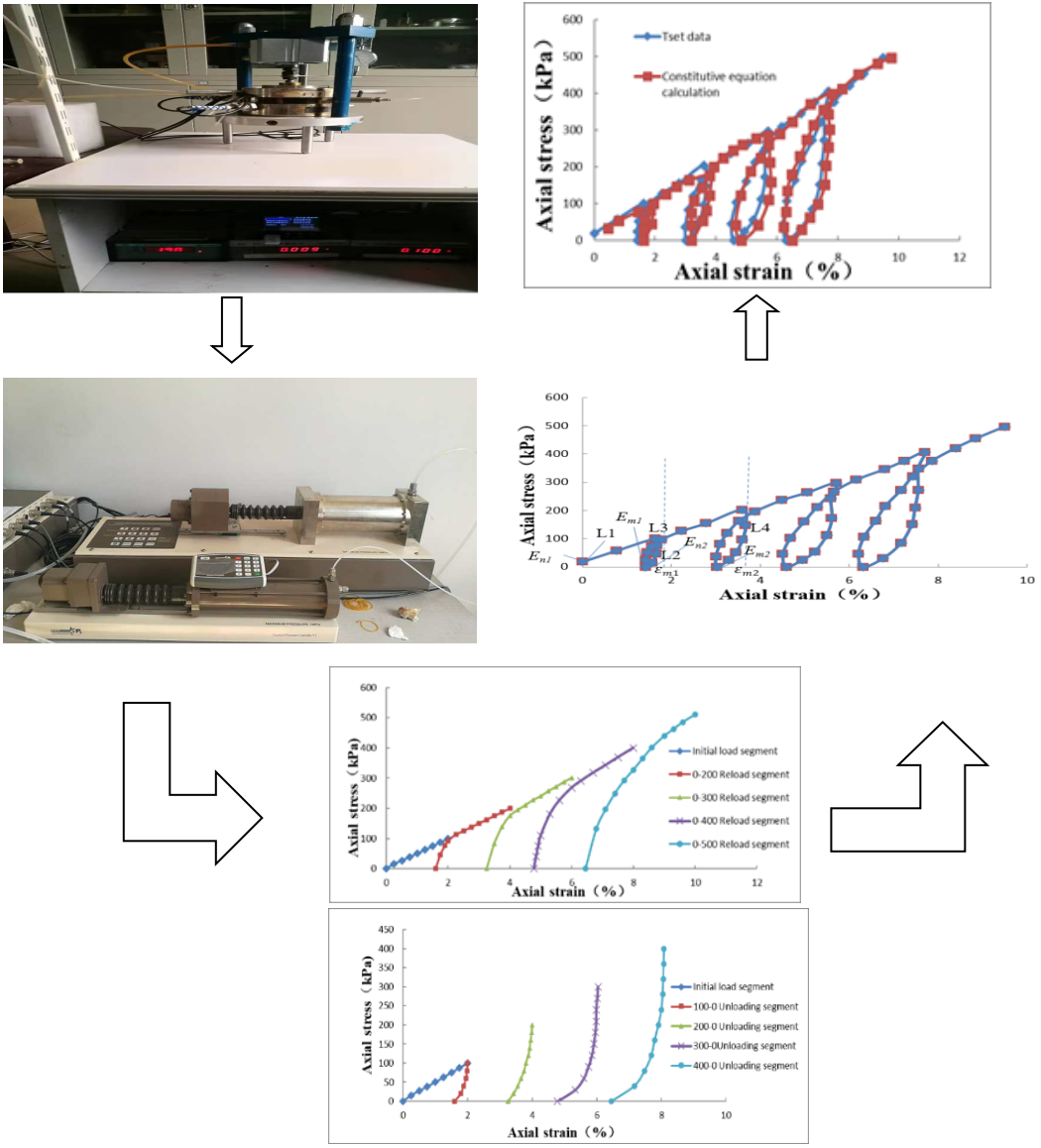#### Highlights

• Described a continuous load one-dimensional K0 consolidation apparatus developed and improved.
• This provides a certain engineering practice value for the strength and deformation characteristics of soft soil under cyclic loading.

## 1. Introduction

With the rapid development of the economy, the construction of transportation infrastructure along the coast and along the Yangtze River has also developed rapidly. More and more ports, airports, highways and railway projects, bridge and tunnel projects, subways and river tunnel projects, underground shopping malls, hydropower stations, and large oil tanks have been built. These projects are often subject to variable loads. Therefore, the unloading effect can be seen, such as tunnel excavation and support, geological tectonic movement, pier foundation rock, etc., which are actually the process of repeated loading and unloading of rock and soil.

Soft clay has obvious rheology. For buildings built on soft soil, the deformation and strength characteristics of soft clay are closely related to its stress state and loading history. Compared with the common uniaxial and triaxial tests, the loading and unloading test can reveal more mechanical phenomena. At present, there are many studies on the deformation characteristics of rock and soil under cyclic loading, while the one-dimensional consolidation axial cyclic loading and unloading tests under ${K}_{0}$ state are still less [1, 2]. Therefore, correct understanding of the strength and deformation characteristics of soft soil under cyclic loading has a certain engineering practice value [3, 4].

In this paper, through the self-developed continuous loading ${K}_{0}$ consolidation instrument, a cyclic loading and unloading test of a certain depth of saturated soil was carried out, and the deformation characteristics of saturated soft clay under cyclic loading, unloading and reloading conditions were analyzed. The relationship between soil elasticity, plastic deformation and stress level during the loading and unloading process is established, and the corresponding expression is established to facilitate the practical application of the project. Finally, based on the experimental results, the cyclic loading and unloading constitutive equation was established [2, 5, 6]; and the plastic hysteresis energy was calculated and fitted. It provides a theoretical basis for deformation prediction of saturated clay foundation, and provides guidance for practical application of engineering.

The test uses soft soil, which is gray, soft and plastic, and has a large amount of organic matter. According to indoor geotechnical tests, the sample height is 40 mm, and the cylinder diameter is 61.8 mm, the plastic limit of the sample is ${w}_{p}=$ 31, and the liquid limit is ${w}_{l}=$ 59, plastic index ${I}_{p}=$ 28. This sample was saturated with vacuum saturated gas. After saturation, the saturation level of soil sample was ${S}_{r}=$ 99 %, $\rho =$ 1.69 g/cm3, and ${e}_{0}=$ 1.44. After the sample is saturated, the cyclic loading and unloading test is performed. The test is made as per the “load-unload-reload” principle. The test has 5 levels of incremental loading and unloading, as shown in Table 1.

## 3. Test results and analysis

### 3.1. Unloading curve analysis of ${\mathbit{K}}_{0}$ – consolidated saturated clay

The axial stress and strain of ${K}_{0}$ – consolidated saturated clay in the unloading conditions at all levels is shown in Fig. 1. The test results show that the change trend of the unloading curves at all levels is the same. The trend of the initial unloading curve is steep, which can be approximated as a straight line. while unloading time is increasing, the unloading is in the form of a curve. The unloading curve is mainly composed of curved segments, which also explains the non-linear characteristics of saturated soft soil, which is different from the rock unloading curve. The deformation modulus of saturated soft soil and the plastic soil deformation of the soil can be obtained by the unloading curve. The surrounding rock of the tunnel is in the unloading environment for a long time. By obtaining the unloading curve in the ${K}_{0}$ – consolidated, the deformation modulus of unloading curve used in modeling calculation is closer to the actual project.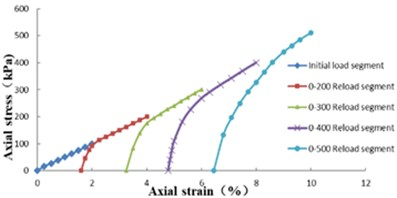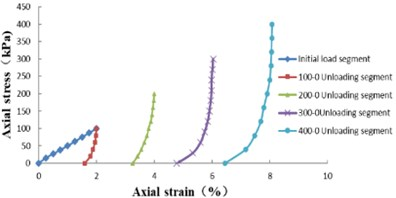### 3.2. Reloading curve analysis of ${\mathbit{K}}_{0}$ – consolidated saturated clay

The axial stress and strain of ${K}_{0}$ – consolidated saturated clay in the Reloading conditions at all levels is shown in Fig. 2. Compared with Fig. 1, the reloading curve and the unloading curve are not caused by the soil plasticity. From the point of view of the reloading curve, the reloading curve approximates a curve before rising to the load of the upper unloading curve. When the load exceeds the load of the upper unloading curve, the reloading curve approximates a straight line and rises along the initial load curve. The loading-unloading curves of unsaturated soils and general foundation soils are basically the same or similar to the initial tangent slope of the initial loading curve, but the slope of the loading-unloading curves of saturated soft soils studied in this experiment is quite different, mainly because the loading-unloading process has a great influence on the physical and mechanical properties of saturated soft soils. The result also shows that the elastic deformation of saturated soft soils is relatively small. In general, the tangent slope of the reloading curve is used to represent the deformation modulus of the reloading curves at all levels, and the deformation characteristics of the reloading curve are described by the average value of the deformation modulus of the reloading curves at all levels .

During the operation of oil tank, the foundation soil was in the state of cyclic loading and unloading, and when the pressure applied in the tank was less than the initial stress of the foundation soil, the modulus of deformation of the reloading curve under ${K}_{0}$ – consolidated was closer to the actual situation when calculating and selecting parameters.

In this work, two-stage cyclic loading and unloading are described, as shown in Fig. 3. It can be seen that ${L}_{1}$ is the initial loading curve, ${L}_{2}$ is the unloading curve, ${L}_{3}$ is the reloading curve, ${L}_{4}$ is the second stage unloading curve, and the initial deformation modulus corresponding to the four curves is ${E}_{n1}$, ${E}_{m2}$, ${E}_{n2}$, ${E}_{m1}$; unloading vertices are $A$ (${\epsilon }_{1}$,${\sigma }_{1}$) and $B$ (${\epsilon }_{2}$,${\sigma }_{2}$), and ${\epsilon }_{p1}$ and ${\epsilon }_{p2}$ are the strains corresponding to zero axial stress during unloading, i.e., the plastic deformation of the sample; ${\sigma }_{n1}$ is the maximum axial stress when the axial strain tends to infinity in the loading stage, and ${\epsilon }_{m1}$ and ${\epsilon }_{m2}$ are the maximum axial strains when the axial stress of the unloading curve tends to infinity. The loading curve ${l}_{1}$ equation can be expressed by hyperbolic equation as follows:

1
${E}_{n}=\frac{\partial \sigma }{\partial \epsilon }=\frac{{E}_{n1}{\sigma }_{n1}^{2}}{{\left({\sigma }_{n1}+{E}_{n1}\epsilon \right)}^{2}}.$

The equation of the unloading curve ${l}_{2}$ can be written as follows:

2
$\sigma =\frac{{E}_{m1}\left(\epsilon -{\epsilon }_{p1}\right)}{1-\left(\frac{\epsilon -{\epsilon }_{p1}}{{\epsilon }_{m1}-{\epsilon }_{p1}}\right)}.$

3
${E}_{m}=\frac{\partial \sigma }{\partial \epsilon }=\frac{{E}_{m1}}{{\left(1-\frac{\epsilon -{\epsilon }_{p1}}{{\epsilon }_{m1}-{\epsilon }_{p1}}\right)}^{2}}.$

According to the same method, the expressions of stress and modulus of deformation can be obtained as follows:

4
$\sigma =\frac{{E}_{n2}{\sigma }_{n2}\left(\epsilon -{\epsilon }_{p1}\right)}{{\sigma }_{n2}+{E}_{n2}\left(\epsilon -{\epsilon }_{p1}\right)},$
5
${E}_{n}=\frac{{E}_{n2}{\sigma }_{n2}^{2}}{{\left[{\sigma }_{n2}+{E}_{n2}\left(\epsilon -{\epsilon }_{p1}\right)\right]}^{2}}.$

According to the above method, the stress-strain equation and deformation modulus of saturated soft soil under cyclic loading and unloading can be expressed as follows.

6
$\sigma =\frac{{E}_{n1}{\sigma }_{n1}\epsilon }{{\sigma }_{n1}+{E}_{n1}\epsilon }.$

7
$\sigma =\frac{{E}_{mi}\left(\epsilon -{\epsilon }_{p\left(i-1\right)}\right)}{1-\frac{\epsilon -{\epsilon }_{p\left(i-1\right)}}{{\epsilon }_{mi}-{\epsilon }_{p\left(i-1\right)}}},$
8
${E}_{m}=\frac{{E}_{mi}}{{\left(1-\frac{\epsilon -{\epsilon }_{p\left(i-1\right)}}{{\epsilon }_{mi}-{\epsilon }_{p\left(i-1\right)}}\right)}^{2}}.$

9
$\sigma =\frac{{E}_{n\left(i+1\right)}{\sigma }_{n\left(i+1\right)}\left(\epsilon -{\epsilon }_{p\left(i-1\right)}\right)}{{\sigma }_{n\left(i+1\right)}+{E}_{n\left(i+1\right)}\left(\epsilon -{\epsilon }_{p\left(i-1\right)}\right)},$
10
${E}_{n}=\frac{{E}_{ni}{\sigma }_{ni}^{2}}{{\left[{\sigma }_{ni}+{E}_{ni}\left(\epsilon -{\epsilon }_{p\left(i-1\right)}\right)\right]}^{2}}.$

In which, 1, 2,…, $n\text{.}$ Eqs. (6-10) contain the constitutive equations of cyclic loading-unloading curve and the expression of deformation modulus of saturated soft soil. The parameters in the formula can be obtained from cyclic loading-unloading test in conventional consolidation apparatus. The axial strain at a certain time can be calculated by applying the applied axial stress.

Fig. 3Axial stress and axial strain diagram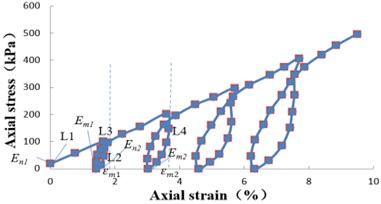### 3.3.2. Verification of constitutive equation

By substituting the parameters in Table 2 into Eqs. (6), (7), (9), the axial strain corresponding to arbitrary axial stress under cyclic loading and unloading can be obtained. The constitutive equation calculation and experimental data are compared and analyzed, as shown in Fig. 4.

Fig. 4Constitutive equation calculation and test data contrast – analysis diagram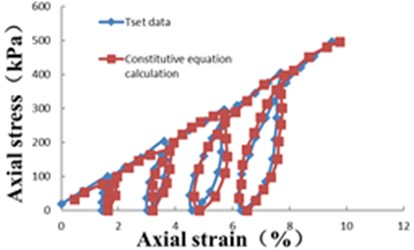From Fig. 4, it can be seen that there are some differences between the calculated results and the test results, but the overall trend is the same, that shows that the constitutive equation can be used to describe the cyclic loading and unloading characteristics of saturated soft soil.

Table 2Constitutive equation parameters

 Loading (KPa) Loading curve Unloading curve ${E}_{ni}$ ${\sigma }_{ni}$ (KPa) ${E}_{mi}$ ${\epsilon }_{mi}$ (%) ${\epsilon }_{p\left(i-1\right)}$ (%) 100 88.5 193 67.8 2.14 1.597 200 370.4 227 57.3 4.33 3.195 300 476.2 345 36.5 6.33 4.723 400 555.5 477 35.7 8.52 6.385 500 588.2 625 – – –

## 4. Conclusions

Based on the analysis of the test data of saturated soft soil under axial cyclic loading and unloading, the following conclusions are drawn: